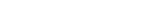MathExamle.com# Positive

Select from the list a generator that creates examples with answers and intermediate calculations that always positive

Creates examples with addition and subtraction operations of integers, the answers are always positive

5 Variants 5 Levels
 14-(2+12)= 12-10= 20-2= 11+(8-6)= 9-(17-9)= 16+(2+2)=
Variant: A Level: Easy

Creates expressions with comparisons of integers, the numbers are always positive

3 Variants 4 Levels
 100-0 > 83-16 3×3 < 72÷3
Variant: A Level: Normal

Creates examples with multiplication and division integers

6 Variants 4 Levels
 9800÷8= 2400÷80= 6888÷3= 2826÷18= 3000÷20= 1026÷6=
Variant: 2 Level: Difficult

Creates examples for addition or subtraction of integers by a column method

3 Variants 3 Levels
 + 883665 116335
 + 120000 352877
 + 374891 205109
Variant: 1 Level: Hard

Creates linear equations, the answers are always positive and integer

4 Variants 3 Levels
 47+x×4=47 18+48÷x=20 x÷5-3=2 x×7+20=90 33+x÷3=56 5×x+40=90
Variant: 3 Level: Normal

Creates examples with a division operation, that can contain the remainder of the division

1 Variant 3 Levels
 29 9
 47 5
 15 3
 27 7
Variant: 1 Level: Easy

Creates examples with a multiplication operation of integers by a column method

1 Variant 5 Levels
 × 5470 2657
 × 4130 4059
 × 9641 4153
Variant: 1 Level: Hard

Creates examples with a division operation of integers by a column method

1 Variant 5 Levels
 22400÷32= 60000÷30= 11473÷11= 49987÷37= 37200÷10= 4725÷25=
Variant: 1 Level: Difficult

Creates examples with operations of addition, subtraction, multiplication and division of integers, the answers are always positive

3 Variants 3 Levels
 687-6×102= 120×6-427= 840÷15+312= 538+13×18=
Variant: 1 Level: Normal

Creates examples with a long division of integers according to the British system

1 Variant 5 Levels
 4 28
 3 99
 9 90
 6 60
Variant: 1 Level: Light

Creates examples with a long division according to the British system, that can contain the remainder of the division

1 Variant 3 Levels
 182÷17= 112÷14= 120÷11= 76÷4= 172÷16= 90÷6=
Variant: 1 Level: Normal

Creates expressions with the operation of rounding integers to tens, hundreds or thousands

3 Variants 3 Levels
 240839≈ 674799≈ 402442≈ 102436≈ 425693≈ 822773≈
Variant: 1 Level: Difficult

### Partitive

Creates examples with addition and subtraction operations of decimals, the answers are always positive

3 Variants 4 Levels
 599.447-549.447= 320.832-72.355+48.523=
Variant: A Level: Hard

Creates examples with a division operation of decimals by a column method

1 Variant 3 Levels
 30.6 8.5
 0.3 0.2
 46.2 2
 48.6 1.2
Variant: 1 Level: Easy

Creates examples with multiplication and division of decimals

5 Variants 4 Levels
 1.1×2.4÷3= 66×1.25×0.08= 1.6÷5×1.5= 5.5×1.8×0.8=
Variant: 4 Level: Normal

Creates expressions with the operation of rounding decimals

3 Variants 3 Levels
 13.2≈ 37.6≈ 86.6≈ 93.1≈ 44.3≈ 11.9≈ 48.1≈ 93.7≈
Variant: 1 Level: Easy

### Numerator

Creates examples with addition and subtraction of fractions, the answers are always positive

3 Variants 3 Levels
 55 + 5 = 6 6
 9 1 – 7 – 4 = 2 10 5
Variant: A Level: Normal

Creates expressions with comparisons of decimals, the numbers are always positive

3 Variants 4 Levels
 80.9-60.7 < 34.9+47.9 99.2-59.5 > 48.2-25.8
Variant: 2 Level: Easy

Creates expressions with comparisons of fractions, the numbers are always positive

3 Variants 3 Levels
 27 > 10 28 13
Variant: A Level: Normal

Creates examples with a long division of decimals according to the British system

1 Variant 3 Levels
 0.46÷0.02= 0.9÷1.2= 12÷3.2= 27.36÷0.36= 14.4÷2.4= 0.38÷0.02=
Variant: 1 Level: Normal

Creates examples with a division operation of integers, the answers may contain a decimal point

1 Variant 3 Levels
 73÷25= 11÷2= 74÷8= 71÷5= 36÷3= 76÷8=
Variant: 1 Level: Normal

Creates examples with operations of addition, subtraction, multiplication and division of decimals, the answers are always positive

3 Variants 4 Levels
 52.92÷1.47-19= 88.81-2.2÷27.5-66.4= 99.8+19.6÷98= 6.88×5+1.04×15=
Variant: A Level: Normal

Creates examples with multiplication and division of fractions

3 Variants 3 Levels
 7 × 17 2 = 11 7
 1 × 26 8 = 2 29
Variant: 1 Level: Normal

Creates expressions with fractions which need to reduce

1 Variant 3 Levels
 4 = 6
 20 = 8
 132 = 30
Variant: 1 Level: Easy

Creates examples with operations of addition, subtraction, multiplication and division of fractions, the answers are always positive

2 Variants 3 Levels
 8 3 ÷ 7 – 4 2 = 4 8 3
 2 1 + 1 × 1 = 5 2 10
Variant: 1 Level: Easy

Creates examples with a long division of integers according to the British system, the answers may contain a decimal point

1 Variant 3 Levels
 4 24
 40 96
 24 63
Variant: 1 Level: Difficult

Creates system of equations, the answers are always integers and positive

3 Variants 3 Levels
 x+y=800 y-x=380
Variant: A Level: Difficult